4.3 Stacks and Queues

# 4.3 Stacks and Queues

In this section, we introduce two closely-related data types for manipulating arbitrarily large collections of objects: the stack and the queue. Each is defined by two basic operations: insert a new item, and remove an item. When we insert an item, our intent is clear. But when we remove an item, which one do we choose? The rule used for a queue is to always remove the item that has been in the collection the most amount of time. This policy is known as first-in-first-out or FIFO. The rule used for a stack is to always remove the item that has been in the collection the least amount of time. This policy is known as last-in first-out or LIFO.

## Pushdown StacksA pushdown stack (or just a stack) is a collection that is based on the last-in-first-out (LIFO) policy. When you click a hyperlink, your browser displays the new page (and inserts it onto a stack). You can keep clicking on hyperlinks to visit new pages. You can always revisit the previous page by clicking the back button (remove it from a stack). The last-in-first-out policy offered by a pushdown stack provides just the behavior that you expect.

By tradition, we name the stack insert operation push and the stack remove operation pop. We also include a method to test whether the stack is empty. The following API summarizes the operations: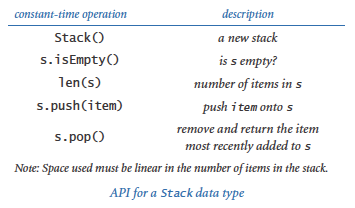## Python List (Resizing Array) Implementation of a Stack

Representing a stack with a Python list is a natural idea, but before reading further, it is worthwhile for you to think for a moment about how you would do so.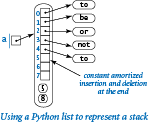Naturally, you need an instance variable `a[]` to hold the stack items in a Python list. For efficiency, we store the items in order of their insertion because inserting and deleting from the end of a Python list takes constant amortized time per operation (whereas inserting and deleting from the front takes linear time per operation).

We could hardly hope for a simpler implementation of the Stack API than arraystack.py — all of the methods are one-liners! The instance variable is a Python list `_a[]` that hold the items in the stack in order of their insertion. To push an item, we append it to the end of the list using the `+=` operator; to pop an item, we call the `pop()` method, which removes and returns the item from the end of the list; to determine the size of the stack, we call the built-in `len()` function. These operations preserve the following properties:

• The stack contains `len(_a)` items.
• The stack is empty when `len(_a)` is 0.
• The list `_a[]` contains the stack items, in order of their insertion.
• The item most recently inserted onto the stack (if nonempty) is `_a[len(_a) - 1]`.

The test client in arraystack.py allows for testing with an arbitrary sequence of operations: it does a `push()` for each string on standard input except the string consisting of a minus sign, for which it does a `pop()`. The diagram at right is a trace for the test file tobe.txt.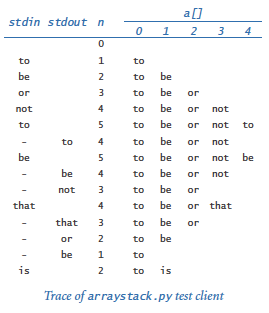The primary characteristics of this implementation are that it uses space linear in the number of items in the stack and that the the push and pop operations take constant amortized time.

## Linked-List Implementation of a Stack

Next, we consider a completely different way to implement a stack, using a fundamental data structure known as a linked list. Reuse of the word "list" here is a bit confusing, but we have no choice — linked lists have been around much longer than Python.

A linked list is a recursive data structure defined as follows: it is either empty (null) or a reference to a node having a reference to a linked list. The node in this definition is an abstract entity that might hold any kind of data, in addition to the node reference that characterizes its role in building linked lists.

With object-oriented programming, implementing linked lists is not difficult. We start with a class for the node abstraction:

 ```class Node: def __init__(self, item, next): self.item = item self.next = next ```

An object of type `Node` has two instance variables: `item` (a reference to an item) and `next` (a reference to another `Node` object). The next instance variable characterizes the linked nature of the data structure. To emphasize that we are just using the Node class to structure the data, we define no methods other than the constructor. We also omit leading underscores from the names of the instance variables, thus indicating that it is permissible for code external to the data type (but still within our Stack implementation) to access those instance variables.

Now, from the recursive definition, we can represent a linked list with a reference to a `Node` object, which contains a reference to an item and a reference to another `Node` object, which contains a reference to an item and a reference to another `Node` object, and so forth. The final `Node` object in the linked list must indicate that it is, indeed, the final Node object. In Python, we accomplish that by assigning `None` to the next instance variable of the final `Node` object. Recall that `None` is a Python keyword — a variable assigned the value `None` references no object.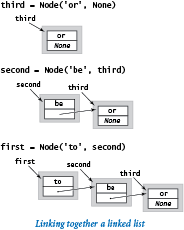For example, to build a linked list that contains the items `'to'`, `'be'`, and `'or'`, we execute this code:

 ```third = Node('or', None) second = Node('be', third) first = Node('to', second) ```

For economy, we use the term link to refer to a `Node` reference. For simplicity, when the item is a string (as in our examples), we put it within the node rectangle (rather than using the more accurate rendition in which the node holds a reference to a string object, which is external to the node). This visual representation allows us to focus on the links.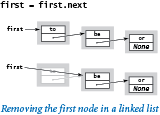Suppose that you want to remove the first node from a linked list. This operation is easy: simply assign to `first` the value `first.next`. Normally, you would retrieve the item (by assigning it to some variable) before doing this assignment, because once you change the variable `first`, you may lose any access to the node to which it was referring previously. Typically, the `Node` object becomes an orphan, and Python's memory management system eventually reclaims it.Now, suppose that you want to insert a new node into a linked list. The easiest place to do so is at the beginning of the linked list. For example, to insert the string `'not'` at the beginning of a given linked list whose first node is `first`, we save `first` in a variable `oldFirst`; create a new `Node` whose item instance variable is `'not'` and whose `next` instance variable is `oldFirst`; and assign first to refer to that new `Node`.

Those two operations take constant time; their efficiency is independent of the length of the list.

### Implementing stacks with linked lists.Program linkedstack.py uses a linked list to implement a stack. The implementation is based on a private `_Node` class that is identical to the `Node` class that we have been using. We make the class private because clients of the `Stack` data type do not need to know any of the details of the linked lists. As usual, we give the class a name that begins with a leading underscore to emphasize to `Stack` clients that they should not access the `_Node` class directly.

### Linked list traversal.

Many linked-list applications need to iterate over the items in a linked list. To do so, we first initialize a loop index variable `cur` that references the first `Node` of the linked list. Next, we get the item associated with `cur` by accessing `cur.item`, and then update `cur` to refer to the next `Node` in the linked list, assigning to it the value of `cur.next` and repeating this process until `cur` is `None` (which indicates that we have reached the end of the linked list). This process is known as traversing the list. The `__str__()` method defined in linkedstack.py does a list traversal.

For stacks, linked lists are significant because they allow us to implement the `push()` and `pop()` methods in constant time in the worst case, while using only a small constant factor of extra space (for the links). Still, Python programmers usually prefer Python lists (resizing arrays), primarily because of the substantial Python overhead for user-defined types like our linked-list `Node`.

## Stack Applications

Pushdown stacks play an essential role in computation. Some examples illustrate.

### Arithmetic expressions.

Some of the first programs that we considered in Chapter 1 involved computing the value of arithmetic expressions like this one:
 ```( 1 + ( ( 2 + 3 ) * ( 4 * 5 ) ) ) ```

How does Python do this calculation? We can address the essential ideas just by composing a Python program that can take a string as input (the expression) and produce the number represented by the expression as output. For simplicity, we begin with the following explicit recursive definition: an arithmetic expression is either a number or a left parenthesis followed by an arithmetic expression followed by an operator followed by another arithmetic expression followed by a right parenthesis. For simplicity, this definition applies to fully parenthesized arithmetic expressions, which specifies precisely which operators apply to which operands. For specificity, we support the familiar binary operators *, +, and -, as well as a square-root operator `sqrt` that takes only one argument.

### Arithmetic expression evaluation.

Precisely how can we convert an arithmetic expression — a string of characters — to the value that it represents? A remarkably simple algorithm that was developed by Edsgar Dijkstra in the 1960s uses two pushdown stacks (one for operands and one for operators) to do this job. An expression consists of parentheses, operators, and operands (numbers). Proceeding from left to right and taking these entities one at a time, we manipulate the stacks according to four possible cases, as follows:

• Push operands onto the operand stack.
• Push operators onto the operator stack.
• Ignore left parentheses.
• On encountering a right parenthesis, pop an operator, pop the requisite number of operands, and push onto the operand stack the result of applying that operator to those operands.

After the final right parenthesis has been processed, there is one value on the stack, which is the value of the expression. The program evaluate.py is an implementation of this algorithm. Try running it with expression1.txt and expression2.txt.

### Stack-based programming languages.

Remarkably, Dijkstra's two-stack algorithm also computes the same value as in our example for this expression:
 ```( 1 ( ( 2 3 + ) ( 4 5 * ) * ) + ) ```

In other words, we can put each operator after its two operands instead of between them. In such an expression, each right parenthesis immediately follows an operator so we can ignore both kinds of parentheses, writing the expressions as follows:

 ```1 2 3 + 4 5 * * + ```

This notation is known as reverse Polish notation, or postfix. To evaluate a postfix expression, we use one stack. Proceeding from left to right, taking these entities one at a time, we manipulate the stacks according to just two possible cases:

• Push operands onto the operand stack.
• On encountering an operator, pop the requisite number of operands and push onto the operand stack the result of applying the operator to those operands.

Again, this process leaves one value on the stack, which is the value of the expression. This representation is so simple that some programming languages, such as Forth (a scientific programming language) and PostScript (a page description language that is used on most printers) use explicit stacks.

### Function-call abstraction.

When the flow of control enters a function, Python creates the function's parameter variables on top of the other variables that might already exist. As the function executes, Python creates the function's local variables — again on top of the other variables that might already exist. When flow of control returns from a function, Python destroys that function's local and parameter variables. In that sense Python creates and destroys parameter and local variables in stack-like fashion. Indeed, most programs use stacks implicitly because they support a natural way to implement function calls

## FIFO Queues

A FIFO queue (or just a queue) is a collection that is based on the first-in first-out (FIFO) policy. Queues are a natural model for so many everyday phenomena that their properties were studied in detail even before the advent of computers.

As usual, we begin by articulating an API. Again by tradition, we name the queue insert operation enqueue and the remove operation dequeue, as indicated in the API below.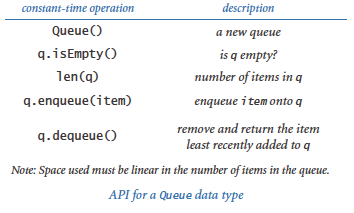Applying our knowledge from stacks, we can use either Python lists (resizing arrays) or linked lists to develop implementations where the operations take constant time and the memory associated with the queue grows and shrinks with the number of elements in the queue.

To implement a queue with a linked list, we keep the items in order of their arrival (the reverse of the order that we used in linkedstack.py). The implementation of `dequeue()` is the same as the `pop()` implementation in linkedstack.py (save the item in the first node, remove the first node from the queue, and return the saved item). Implementing `enqueue()`, however, is a bit more challenging: how do we add a node to the end of a linked list? To do so, we need a link to the last node in the list, because that node's link has to be changed to reference a new node containing the item to be inserted. So we maintain a second instance variable that always references the last node in the linked list.

The program linkedqueue.py is a linked-list implementation of `Queue` that has the same performance properties as `Stack`: all of the methods are constant-time operations, and space usage is linear in the number of items on the queue.

### Resizing array implementation.

It is also possible to develop a FIFO queue implementation that is based on an explicit resizing array representation that has the same performance characteristics as those that we developed for a stack in arraystack.py. This implementation is a worthy and classic programming exercise that you are encouraged to pursue further in the exercises at the end of this section. It might be tempting to use one-line calls on Python `list` methods, as in arraystack.py. However, the methods for inserting or deleting items at the front of a Python list will not fill the bill, as they take linear time.

### Random queues.

Even though they are widely applicable, there is nothing sacred about the FIFO and LIFO disciplines. It makes perfect sense to consider other rules for removing items. One of the most important to consider is a data type for which `dequeue()` removes a random item (sampling without replacement) and `sample()` returns a random item without removing it from the queue (sampling with replacement). Such actions are precisely called for in numerous applications, some of which we have already considered, starting with sample.py from Section 1.4. With a Python list (resizing array) representation, implementing `sample()` is straightforward, and we can use the same idea as in sample.py to implement `dequeue()` (exchange a random item with the last item before removing it). We use the name `RandomQueue` to refer to this data type (see the "Random queue" creative exercise at the end of this section).

## Queue Applications

In the past century, FIFO queues proved to be accurate and useful models in a broad variety of applications. A field of mathematics known as queuing theory has been used with great success to help understand and control complex systems of all kinds. Understanding and controlling such a complex system involves solid implementations of the queue abstraction, application of mathematical results of queueing theory, and simulation studies involving both. We consider next a classic example to give a flavor of this process.

### M/M/1 queue.

One of the most important queueing models is known as an M/M/1 queue, which has been shown to accurately model many real-world situations, such as a single line of cars entering a toll booth or patients entering an emergency room. The M stands for Markovian or memoryless and indicates that both arrivals and services are Poisson processes: both the interarrival times and service times obey an exponential distribution (see Exercise 2.2.12) and the 1 indicates that there is one server. An M/M/1 queue is parameterized by its arrival rate λ (for example, the number of cars per minute arriving at the toll booth) and its service rate μ (for example, the number of cars per minute that can pass through the toll booth) and is characterized by three properties:

• There is one server — a FIFO queue.
• Interarrival times to a queue obey an exponential distribution with rate λ per minute.
• Service times from a nonempty queue obey an exponential distribution with rate μ per minute.

The average time between arrivals is 1/λ minutes and the average time between services (when the queue is nonempty) is 1/μ minutes. So, the queue will grow without bound unless μ > λ; otherwise, customers enter and leave the queue in an interesting dynamic process.

In practical applications, people are interested in the effect of the parameters λ and μ on various properties of the queue. For M/M/1 queues, it is known that the average number of customers in the system L is λ / (μ - λ), and the average time a customer spends in the system W is 1 / (μ - λ). These formulas confirm that the wait time (and queue length) grows without bound as λ approaches μ. They also obey a general rule known as Little's law: the average number of customers in the system is λ times the average time a customer spends in the system (L - λW) for many types of queues.

The program mm1queue.py is a `Queue` client that you can use to validate these sorts of mathematical results. It is a simple example of an event-based simulation: we generate events that take place at particular times and adjust our data structures accordingly for the events, simulating what happens at the time they occur. See the textbook for details. From a practical point of view, one of the most important characteristics of the process, which you can discover for yourself by running mm1queue.py for various values of the parameters λ and μ, is that the average time a customer spends in the system (and the average number of customers in the system) can increase dramatically when the service rate approaches the arrival rate.

 `% python mm1queue.py .167 .25` `% python mm1queue.py .167 .20`### Resource allocation.

A resource-sharing system involves a large number of loosely cooperating servers that want to share resources. Each server agrees to maintain a queue of items for sharing, and a central authority distributes the items to the servers (and informs users where they may be found). We will consider the kind of program that the central authority might use to distribute the items, ignoring the dynamics of deleting items from the systems, adding and deleting servers, and so forth.

Central authorities often use a random policy, where the assignments are based on random choice. An even better policy is to choose a random sample of servers and assign a new item to the one that has smallest number of items. But how big a sample should we take?

The program loadbalance.py is a simulation of the sampling policy, which we can use to study this question. This program makes good use of the `RandomQueue` data type (see the "Random queue" creative exercise at the end of this section) to provide an easily understood program that we can use for experimentation. The simulation maintains a random queue of queues and builds the computation around an inner loop where each new request for service goes on the smallest of a sample of queues, using the `sample()` method from `RandomQueue` to randomly sample queues. The surprising end result is that samples of size 2 lead to near-perfect balancing, so there is no point in taking larger samples.

 `% python loadbalance.py 50 500 1` `% python loadbalance.py 50 500 2`#### Q & A

Q. When should I call the `_Node` constructor?

A. Just as with any other class, you should call the `_Node` constructor when you want to create a new `_Node` object (a new node in the linked list). You should not use it to create a new reference to an existing `_Node` object. For example, the code

 ```oldfirst = _Node(item, next) oldfirst = first ```

creates a new `_Node` object, then immediately loses track of the only reference to it. This code does not result in an error, but it is a bit untidy to create orphans for no reason.

Q. Why not define `Node` as a stand-alone class in a separate file named `node.py`?

A. By defining `_Node` in the same file as linkedstack.py or linkedqueue.py and giving it a name that begins with an underscore, we encourage clients of the `Stack` or `Queue` classes not to use the `_Node` class directly. Our intention is that the `_Node` objects be used only in the linkedstack.py or linkedqueue.py implementations, not in other clients.

Q. Should a client be allowed to insert the item `None` onto a stack or queue?

A. This question arises frequently when implementing collections in Python. Our implementations do permit the insertion of any object, including `None`.

Q. Are there standard Python modules for stacks and queues?

A. Not really. As noted earlier in this section, Python's built-in `list` data type has operations that make it is easy to efficiently implement a stack using a list. But the `list` data type also needs many additional methods that are not normally associated with a stack, such as indexed access and deleting an arbitrary item. The prime advantage of restricting ourselves to the set of operations that we need (and only those operations) is that it makes it easier to develop an implementation that can provide the best possible performance guarantees for those operations. Python also includes a data type `collections.deque` that implements a mutable sequence of items with efficient insertion and deletion to either the front or the back.

Q. Why not have a single data type that implements methods to insert an item, remove the most recently inserted item, remove the least recently inserted item, remove a random item, iterate over the items, return the number of items in the collection, and whatever other operations we might desire? Then we could get them all implemented in a single class that could be used by many clien

ts.

A. This is an example of a wide interface, which, as we pointed out in Section 3.3, should be avoided. As just mentioned, one reason to avoid wide interfaces is that it is difficult to construct implementations that are efficient for all operations. A more important reason is that narrow interfaces enforce a certain discipline on your programs, which makes client code much easier to understand. If one client uses `Stack` and another uses `Queue`, we have a good idea that the LIFO discipline is important to the first and the FIFO discipline is important to the second. Another approach is to use inheritance to try to encapsulate operations that are common to all collections. However, such implementations are best left for experts, whereas any programmer can learn to build implementations such as `Stack` and `Queue`.

Q. Is there any way that I can compose a client that uses both arraystack.py and linkedstack.py in the same program?

A. Yes, the easiest way is to add an `as` clause to the `import` statement, as below. In effect, this kind of `import` statement creates an alias for the name of the class and your code can then use that alias instead of the name of the class.

 ```from arraystack import Stack as ArrayStack from linkedstack import Stack as LinkedStack ... stack1 = ArrayStack() stack2 = LinkedStack() ```

#### Exercises

1. Give the output written by arraystack.py for the following input:

 ```it was - the best - of times - - - it was - the - - ```
2. Give the contents and length of the array for arraystack.py after each operation for the following input:

 ```it was - the best - of times - - - it was - the - - ```
3. Suppose that a client performs an intermixed sequence of push and pop operations on a `Stack`. The push operations put the integers 0 through 9 in order onto the stack; the pop operations write the return value. Which of the following sequence(s) could not occur?

1. `4 3 2 1 0 9 8 7 6 5`
2. `4 6 8 7 5 3 2 9 0 1`
3. `2 5 6 7 4 8 9 3 1 0`
4. `4 3 2 1 0 5 6 7 8 9`
5. `1 2 3 4 5 6 9 8 7 0`
6. `0 4 6 5 3 8 1 7 2 9`
7. `1 4 7 9 8 6 5 3 0 2`
8. `2 1 4 3 6 5 8 7 9 0`
4. Compose a stack client `reverse.py` that reads in strings from standard input and writes them in reverse order to standard output. Compose a stack client `parentheses.py` that reads in a text stream from standard input and uses a stack to determine whether its parentheses are properly balanced. For example, your program should write `True` for `[()]{}{[()()]()}` and `False` for `[(])`.

5. Add the method `__len__()` to the `Stack` class in linkedstack.py.

6. Add a method `peek()` to the `Stack` class in arraystack.py that returns the most recently inserted item on the stack (without popping it).

7. What does the following code fragment write when `n` is 50? Give a high-level description of what the code fragment does for a given positive integer `n`.

 ```stack = Stack() while n > 0: stack.push(n % 2) n /= 2 while not stack.isEmpty(): stdio.write(stack.pop()) stdio.writeln() ```

Solution: It writes the binary representation of `n` (`110010` when `n` is 50).

8. What does the following code fragment do to the queue `queue`?

 ```stack = Stack() while not queue.isEmpty(): stack.push(queue.dequeue()) while not stack.isEmpty(): queue.enqueue(stack.pop()) ```
9. Draw an object-level trace diagram for the three-node example used to introduce linked lists in this section.

10. Compose a program that takes from standard input an expression without left parentheses and writes the equivalent infix expression with the parentheses inserted. For example, given the input

 ```1 + 2 ) * 3 - 4 ) * 5 - 6 ) ) ) ```

your program should write

 ```( ( 1 + 2 ) * ( ( 3 - 4 ) * ( 5 - 6 ) ) ```
11. Compose a filter `infixtopostfix.py` that converts a fully parenthesized arithmetic expression from infix to postfix.

12. Compose a program `evaluatepostfix.py` that reads a postfix expression from standard input, evaluates it, and writes the value to standard output. (Piping the output of your program from the previous exercise to this program gives equivalent behavior to evaluate.py.)

13. Suppose that a client performs an intermixed sequence of enqueue and dequeue operations on a `Queue`. The enqueue operations put the integers 0 through 9 in order onto the queue; the dequeue operations write the return value. Which of the following sequence(s) could not occur?

1. `0 1 2 3 4 5 6 7 8 9`
2. `4 6 8 7 5 3 2 9 0 1`
3. `2 5 6 7 4 8 9 3 1 0`
4. `4 3 2 1 0 5 6 7 8 9`
14. Compose a `Queue` client that takes a command-line argument `k` writes the `k`th from the last string found on standard input.

15. Give the running time of each operation in the following `Queue` class, where the item least recently inserted is at `_a`.

 ```class Queue: def __init__(self): self._a = [] def isEmpty(self): return len(self._a) == 0 def __len__(self): return len(self._a) def enqueue(self, item): self._a += [item] def dequeue(self): return self._a.pop(0) ```
16. Give the running time of each operation in the following `Queue` class, where the item most recently inserted is at `_a`.

 ```class Queue: def __init__(self): self._a = [] def isEmpty(self): return len(self._a) == 0 def __len__(self): return len(self._a) def enqueue(self, item): self._a.insert(0, item) def dequeue(self): return self._a.pop() ```
17. Modify mm1queue.py to make a program `md1queue.py` that simulates a queue for which the service times are fixed (deterministic) at rate of μ. Verify Little's law empirically for this model.

#### Linked List Exercises

The following exercises are intended to give you experience in working with linked lists. The easiest way to work them is to make drawings using the visual representation described in the text.

1. Suppose `x` is a linked-list node. What is the effect of the following code fragment?

 ```x.next = x.next.next ```

Solution: Deletes from the list the node immediately following `x`.

2. Compose a function `find()` that takes the first node in a linked list and an object `key` as arguments and returns `True` if some node in the list has `key` as its item field, and `False` otherwise.

3. Compose a function `delete()` that takes the first node in a linked list and an integer `k` as arguments and deletes the `k`th element in a linked list, if it exists.

4. Suppose that `x` is a linked-list node. What is the effect of the following code fragment?

 ```t.next = x.next x.next = t ```

Solution: Inserts node `t` immediately after node `x`.

5. Why does the following code fragment not have the same effect as the code fragment in the previous question?

 ```x.next = t t.next = x.next ```

Solution: When it comes time to update `t.next`, `x.next` is no longer the original node following `x`, but is instead `t` itself!

6. Compose a function `removeAfter()` that takes a linked-list node as an argument and removes the node following the given one (and does nothing if the argument or the next field in the argument node is `None`).

7. Compose a function `copy()` that takes a linked-list node as an argument and creates a new linked list with the same sequence of items, without destroying the original linked list.

8. Compose a function `remove()` that takes a linked-list node and an object item as arguments and removes every node in the list whose item is item.

9. Compose a function `listmax()` that takes the first node in a linked list as an argument and returns the value of the maximum item in the list. Assume that the items are comparable, and return `None` if the list is empty.

10. Develop a recursive solution to the previous question.

11. Compose a function that takes the first node in a linked list as an argument and reverses the list, returning the first node in the result.

Iterative solution: To accomplish this task, we maintain references to three consecutive nodes in the linked list: `reverse`, `first`, and `second`. At each iteration, we extract the node `first` from the original linked list and insert it at the beginning of the reversed list. We maintain the invariant that `first` is the first node of what's left of the original list, `second` is the second node of what's left of the original list, and `reverse` is the first node of the resulting reversed list.

 ```def reverse(first): reverse = None while first is not None: second = first.next first.next = reverse reverse = first first = second return reverse ```

When composing code involving linked lists, we must always be careful to properly handle the exceptional cases (when the linked list is empty, when the list has only one or two nodes) and the boundary cases (dealing with the first or last items). This is usually much trickier than handling the normal cases.

12. Compose a recursive function to write the elements of a linked list in reverse order. Do not modify any of the links. Easy: Use quadratic time, constant extra space. Also easy: Use linear time, linear extra space. Not so easy: Develop a divide-and-conquer algorithm that uses linearithmic time and logarithmic extra space.

Quadratic time, constant space solution: We recursively reverse the part of the list starting at the second node, and then carefully append the first element to the end.

 ```def reverse(first): if first is None: return None if first.next is None: return first second = first.next rest = reverse(second) second.next = first first.next = None return rest ```
13. Compose a recursive function to randomly shuffle the elements of a linked list by modifying the links. Easy: Use quadratic time, constant extra space. Not so easy: Develop a divide-and-conquer algorithm that takes linearithmic time and uses logarithmic extra memory. For the "merging" step, see the "Riffle shuffle" creative exercise at the end of Section 1.4.

#### Creative Exercises

1. Deque. A double-ended queue or deque (pronounced "deck") is a combination of a stack and a queue. Compose a class `Deque` that uses a linked list to implement this API: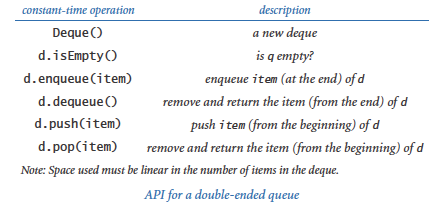2. Josephus problem. In the Josephus problem from antiquity, n people are in dire straits and agree to the following strategy to reduce the population. They arrange themselves in a circle (at positions numbered from 0 to n-1) and proceed around the circle, eliminating every m person until only one person is left. Legend has it that Josephus figured out where to sit to avoid being eliminated. Compose a `Queue` client `josephus.py` that takes n and m from the command line and writes the order in which people are eliminated (and thus would show Josephus where to sit in the circle).

 ```% python josephus.py 7 2 1 3 5 0 4 2 6 ```
3. Merging two sorted queues. Given two queues with strings in ascending order, move all of the strings to a third queue so that the third queue ends up with the strings in ascending order.

4. Nonrecursive mergesort. Given n strings, create n queues, each containing one of the strings. Create a queue of the n queues. Then, repeatedly apply the sorted merging operation to the first two queues and reinsert the merged queue at the end. Repeat until the queue of queues contains only one queue.

5. Delete ith element. Implement a class that supports the following API:First, develop an implementation that uses a Python list (resizing array) implementation, and then develop one that uses a linked-list implementation. (See the "Generalized queue" creative exercise at the end of Section 4.4 for a more efficient implementation that uses a binary search tree.)

6. Queue with two stacks. Show how to implement a queue using two stacks (and only a constant amount of extra memory) so that each queue operations uses a constant amortized number of stack operations.

7. Ring buffer. A ring buffer, or circular queue, is a FIFO data structure of a fixed capacity n. It is useful for transferring data between asynchronous processes or for storing log files. When the buffer is empty, the consumer waits until data is deposited; when the buffer is full, the producer waits to deposit data. Develop an API for a ring buffer and an implementation that uses an array representation (with circular wrap-around).

8. Move-to-front. Read in a sequence of characters from standard input and maintain the characters in a linked list with no duplicates. When you read in a previously unseen character, insert it at the front of the list. When you read in a duplicate character, delete it from the list and reinsert it at the beginning. Name your program `movetofront.py`: it implements the well known move-to-front strategy, which is useful for caching, data compression, and many other applications where items that have been recently accessed are more likely to be reaccessed.

9. Random queue. A random queue stores a collection of items as per this API: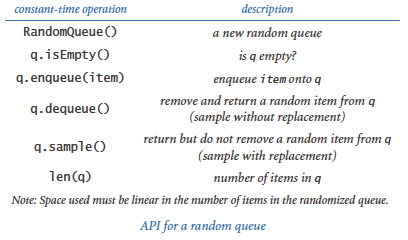Compose a class `RandomQueue` that implements this API. Hint: Use a Python list (resizing array) representation, as in arraystack.py. To remove an item, swap one at a random position (indexed 0 through n-1) with the one at the last position (index n-1). Then delete and return the last object. Compose a client that writes a deck of cards in random order using `RandomQueue`.

Solution: See randomqueue.py.

10. Topological sort. You have to sequence the order of `n` jobs that are numbered from 0 to `n`-1 on a server. Some of the jobs must complete before others can begin. Compose a program `topologicalsorter.py` that takes `n` as a command-line argument and a sequence on standard input of ordered pairs of jobs `i j`, and then writes a sequence of integers such that for each pair `i j` in the input, job `i` appears before job `j`. Use the following algorithm: First, from the input, build, for each job, (1) a queue of the jobs that must follow it and (2) its indegree (the number of jobs that must come before it). Then, build a queue of all nodes whose indegree is 0 and repeatedly delete some job with zero indegree, maintaining all the data structures. This process has many applications; for example, you can use it to model course prerequisites for your major so that you can find a sequence of courses to take so that you can graduate.

11. Text editor buffer. Develop a data type for a buffer in a text editor that implements the following API: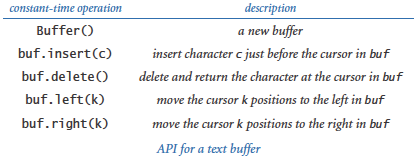Hint: Use two stacks.

12. Copy a stack. Create a `copy()` method for the linked-list implementation of `Stack` so that

 ```stack2 = stack1.copy() ```

makes `stack2` a reference to a new and independent copy of the stack `stack1`. You should be able to push and pop from either `stack1` or `stack2` without influencing the other.

13. Copy a queue. Create a `copy()` method for the linked-list implementation of Queue so that

 ```queue2 = queue1.copy() ```

makes `queue2` a reference to a new and independent copy of the queue `queue1`. Hint: Delete all of the items from `queue1` and add these items to both `queue1` and `queue2`.

14. Stack with explicit resizing array. Implement a stack using an explicit resizing array: initialize an empty stack by using an array of length 1 as an instance variable; double the length of the array when it becomes full and halve the length of the array when it becomes one-fourth full.

Solution:
 ```class Stack: def __init__(self): self._a = [None] self._n = 0 def isEmpty(self): return self._n == 0 def __len__(self): return self._n def _resize(self, capacity): temp = stdarray.create1D(capacity) for i in range(self._n): temp[i] = self._a[i] self._a = temp def push(self, item): if self._n == len(self._a): self._resize(2 * self._n) self._a[self._n] = item self._n += 1 def pop(self): self._n -= 1 item = self._a[self._n] self._a[self._n] = None if (self._n > 0) and (self._n == len(self._a) // 4): self._resize(self._n // 2) return item ```
15. Queue with explicit resizing array. Implement a queue using an explicit resizing array so that all operations take constant amortized time. Hint: The challenge is that the items will "crawl across" the array as items are added to and removed from the queue. Use modular arithmetic to maintain the array indices of the items at the front and back of the queue.

stdin stdout n lo hi a a a a a a a a
0 0 0 None
to 1 0 1 to None
be 2 0 2 to be
or 3 0 3 to be or None
not 4 0 4 to be or not
to 5 0 5 to be or not to None None None
- to 4 1 4 None be or not to None None None
be 5 1 6 None be or not to be None None
- be 4 2 6 None None or not to be None None
- or 3 3 6 None None None not to be None None
that 4 3 7 None None None not to be that None

Solution: See arrayqueue.py.

16. Queue simulations. Study what happens when you modify mm1queue.py to use a stack instead of a queue. Does Little's law hold? Answer the same question for a random queue. Plot histograms and compare the standard deviations of the waiting times.

17. Load-balancing simulations. Revise loadbalance.py to write the average queue length and the maximum queue length instead of plotting the histogram, and use it to run simulations for 1 million items on 100000 queues. Write the average value of the maximum queue length for 100 trials each with sample sizes 1, 2, 3, and 4. Do your experiments validate the conclusion drawn in the text about using a sample of size 2?

18. Listing files. A folder is a list of files and subfolders. Compose a program that takes the name of a folder as a command-line argument and writes all of the file names contained in that folder, with the contents of each folder recursively listed (indented) under that folder's name. Hint: Use a queue, and see the `listdir()` function defined in Python's `os` module.